Test: Inverters- 2

# Test: Inverters- 2 - Electrical Engineering (EE)

Test Description

## 10 Questions MCQ Test GATE Electrical Engineering (EE) 2023 Mock Test Series - Test: Inverters- 2

Test: Inverters- 2 for Electrical Engineering (EE) 2023 is part of GATE Electrical Engineering (EE) 2023 Mock Test Series preparation. The Test: Inverters- 2 questions and answers have been prepared according to the Electrical Engineering (EE) exam syllabus.The Test: Inverters- 2 MCQs are made for Electrical Engineering (EE) 2023 Exam. Find important definitions, questions, notes, meanings, examples, exercises, MCQs and online tests for Test: Inverters- 2 below.
Solutions of Test: Inverters- 2 questions in English are available as part of our GATE Electrical Engineering (EE) 2023 Mock Test Series for Electrical Engineering (EE) & Test: Inverters- 2 solutions in Hindi for GATE Electrical Engineering (EE) 2023 Mock Test Series course. Download more important topics, notes, lectures and mock test series for Electrical Engineering (EE) Exam by signing up for free. Attempt Test: Inverters- 2 | 10 questions in 45 minutes | Mock test for Electrical Engineering (EE) preparation | Free important questions MCQ to study GATE Electrical Engineering (EE) 2023 Mock Test Series for Electrical Engineering (EE) Exam | Download free PDF with solutions
 1 Crore+ students have signed up on EduRev. Have you?
Test: Inverters- 2 - Question 1

### Match List-I (Type of Inverter circuits) with List-II (Applications/Limitations) and select the correct answer using the codes given below the lists: List-I A. Adjustable voltage inverter B. Pulse width modulated inverter C. Current source inverter D. Voltage source inverter List-II 1. Limited speed range of motor 2. Capable of regeneration 3. Good input power factor at al! frequencies 4. Possibility of short circuit across the source Codes: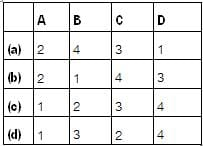Detailed Solution for Test: Inverters- 2 - Question 1

• By using adjustable voltage inverter, speed range of motor is limited because of motor cogging at 6 Hz and below.
• In pulse width modulated inverter, input p.f. is good at all frequencies.
• A CSI has the capability of regeneration back to the a.c. line because d.c. link polarity can be reversed.
• In VSI, the misfiring of switching devices may cause short circuit across the source and create serious problems.

Test: Inverters- 2 - Question 2

### In current source inverters load voltage waveform V0 and load current waveform i0 respectively

Detailed Solution for Test: Inverters- 2 - Question 2

In a CSI, load current rather than load voltage is controlled, and the inverter output voltage is dependent upon the load impedance and the output voltage waveform. The load current and hence its waveform is independent of load impedance due to which a CSI has inherent protection against short-circuit across its terminals.

Test: Inverters- 2 - Question 3

### A single phase IGBT bridge inverter, compared to a single pulse PWM control, multiple pulse PWM

Detailed Solution for Test: Inverters- 2 - Question 3

In case of singte-putse width modulation (PWM), the width of the pulse is adjusted to reduce the harmonic. However, a single phase IGBT bridge inverter produces a square wave. This square wave contains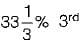harmonic,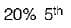harmonic and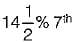harmonic.

Test: Inverters- 2 - Question 4

In a single-pulse modulation of PWM inverters if pulse width is 120° then

Detailed Solution for Test: Inverters- 2 - Question 4

The rms value of amplitude of harmonic voltage of a single, pulse modulated wave is given by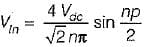(where, p = width of pulse an Vdc = supply dc voltage)
If the 3rd harmonic Is to be eliminated, then
EL3 = 0
i.e.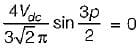or,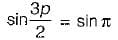or,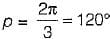= Required pulse width

Test: Inverters- 2 - Question 5

If for a single-phase half-bridge inverter, the amplitude of output voltage is V0 and the output power is P, then their corresponding values for a single-phase full-bridge inverter would be respectively

Detailed Solution for Test: Inverters- 2 - Question 5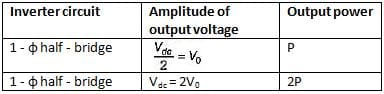Test: Inverters- 2 - Question 6

A single-phase full bridge inverter can operate in load-commutation mode in case load consists of

Detailed Solution for Test: Inverters- 2 - Question 6

In a 1-φ full bridge inverter if RLC load is underdamped, then the two thyristors (namely T1 and T2) shown in figure will get commutated naturally and therefore no commutation circuitry will be needed. Thus, load commutation will be possible.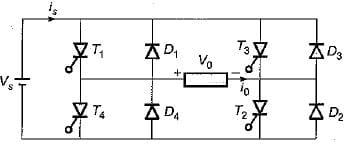Test: Inverters- 2 - Question 7

An inverter has a periodic output voltage with the output waveform as shown in figure.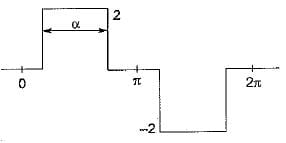When the conduction angle a = 120°, the rms value of the output voltage is

Detailed Solution for Test: Inverters- 2 - Question 7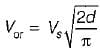(Here, 2 pulse width = 120°)
∴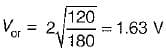Test: Inverters- 2 - Question 8

Assertion (A): The terminal voltage of a voltage source inverter remains substantially constant with variations in load.
Reason (R): Any short-circuit across the terminals of a voltage source inverter causes current to rise very fast.

Detailed Solution for Test: Inverters- 2 - Question 8

A voltage source inverter is one in which the d.c. source has small or negligible impedance. Due to low internal impedance, the terminal voltage of a VSI remains substantially constant with variations in load. Reason is also a correct -statement because due to low time constant of internal impedance any short-circuit across the terminals of a VSI causes current to rise very fast. Hence, both assertion and reason are true but reason is not the correct explanation of assertion.

Test: Inverters- 2 - Question 9

Assertion (A): The lower order harmonics are reduced by using some technique while the higher order harmonics are reduced by using filter.
Reason (R): The cost of the filter is reduced and at the same time transient response is also improved to a great extent.

Test: Inverters- 2 - Question 10

Inverters designed from BJT are preferably used in saturation region than in active region because of

## GATE Electrical Engineering (EE) 2023 Mock Test Series

22 docs|284 tests
Information about Test: Inverters- 2 Page
In this test you can find the Exam questions for Test: Inverters- 2 solved & explained in the simplest way possible. Besides giving Questions and answers for Test: Inverters- 2, EduRev gives you an ample number of Online tests for practice

## GATE Electrical Engineering (EE) 2023 Mock Test Series

22 docs|284 tests(Scan QR code)